Monday 16th September 2019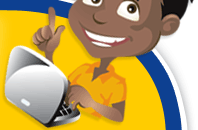# Classroom Key Stage 1 / KS1 - Maths Activities & Resources

## Welcome to the Maths section of the Key Stage 1 classroom. Within KS1 Maths you will find resources, activities and online @school assessment tests that will help children revise and develop the subject of Maths at Key Stage 1 level

•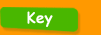•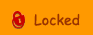•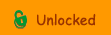••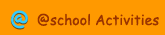•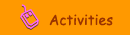•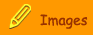###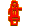Times Tables

0 Times Table - Interactive test for 0 times table.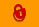1 Times Table - Interactive test for 1 times table.1, 2, 5 and 10 times tables - Interactive activity to learn your times tables.2 5 and 10 Times tables - Interactive test for your 2, 5 and 10 times table - good revision tool.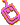2 Times Table - Interactive test for 2 times table.3 Times Table - Interactive test for 3 times table.4 Times Table - Interactive test for 4 times table.5 Times Table - Interactive test for 5 times table.Arrays - Interactive activity to describe arraysConcept of Multiplication - 1 - Interactive activity about understanding multiplication.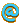Concept of Multiplication - 2 - Interactive activity about understanding multiplication.Cross the Swamp - Use all 4 number operations to help the monkey cross the swampDivision - Interactive activity to learn about divisionFind the Missing Number - Multiplication? - Interactive activity to complete the multiplication calculationsMultiples of 2, 5 and 10 - Interactive activity to recognise multiples of 2, 5 and 10.Number Halving - Interactive activity where you need to find the half of a group of objetcs.Number Quartering - Interactive activity where you need to find a quarter from a group of objects.Times it - Interactive activity for the 2, 5 and 10 times tables